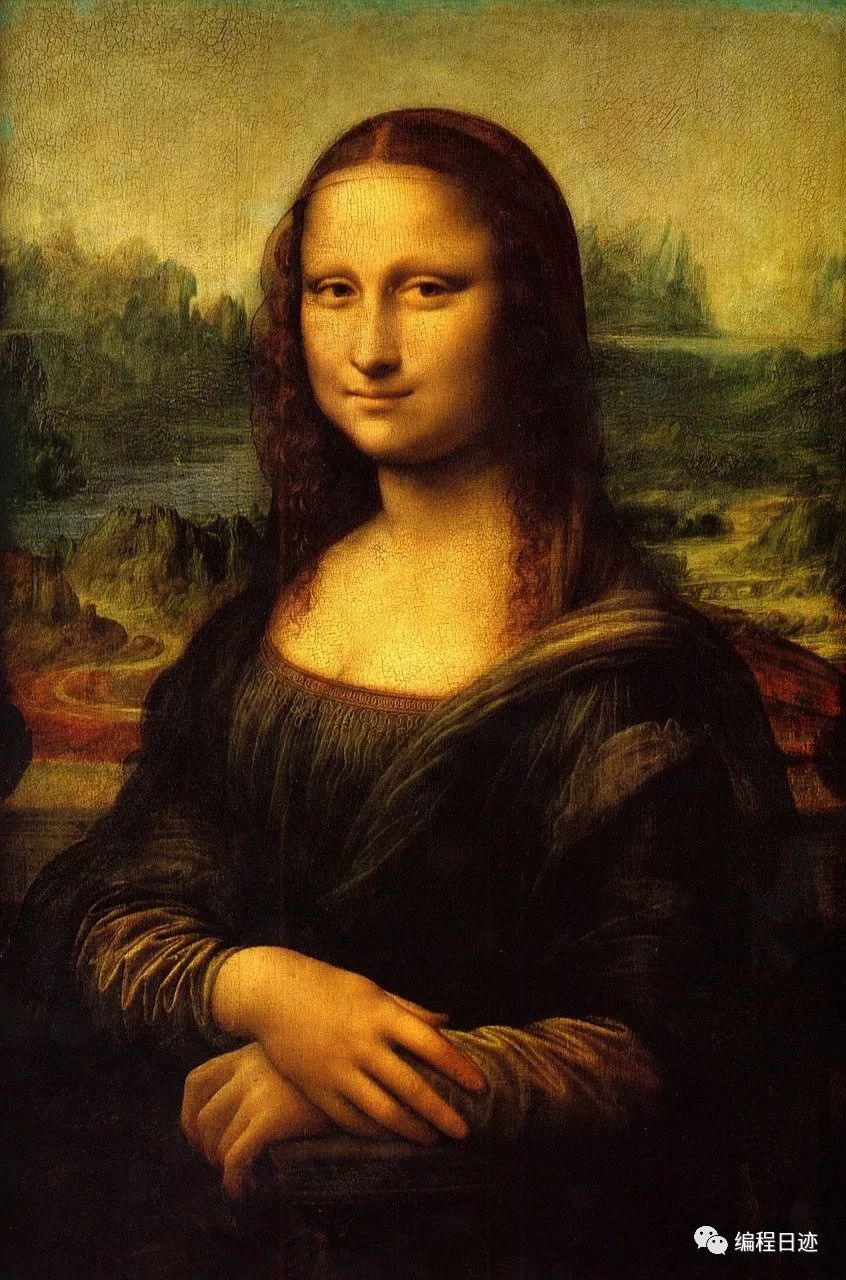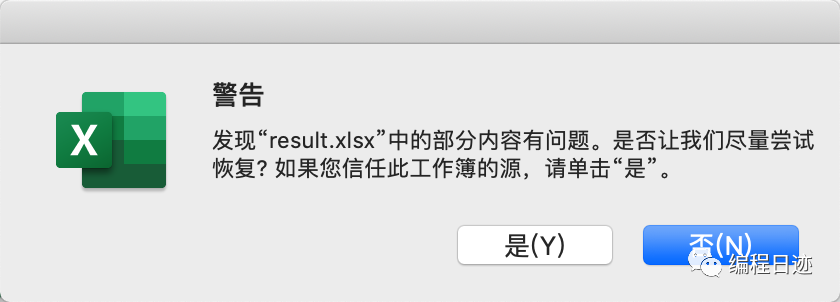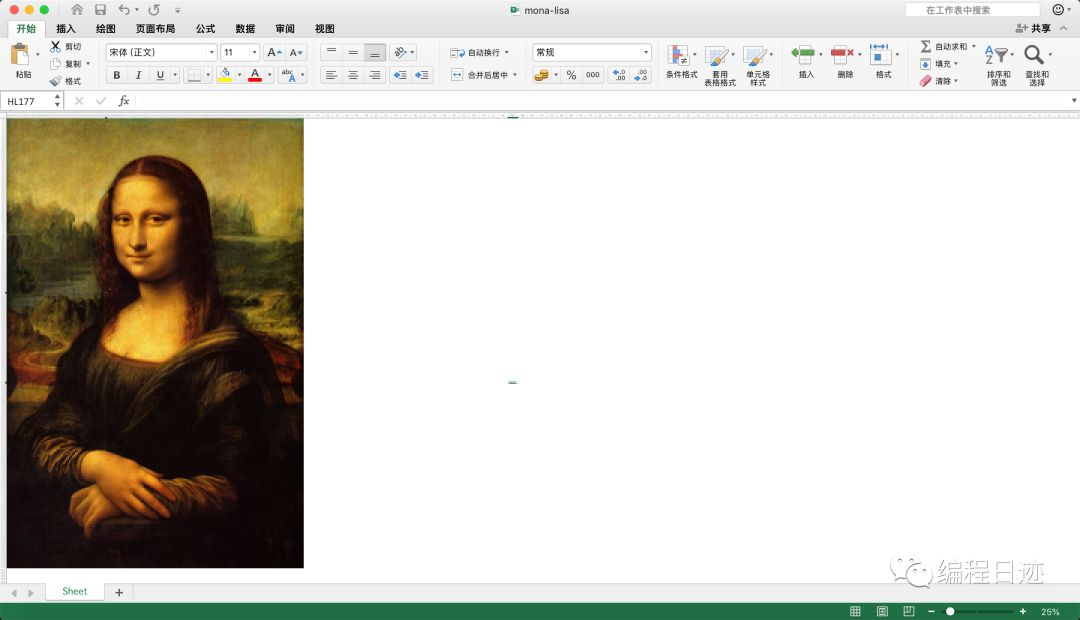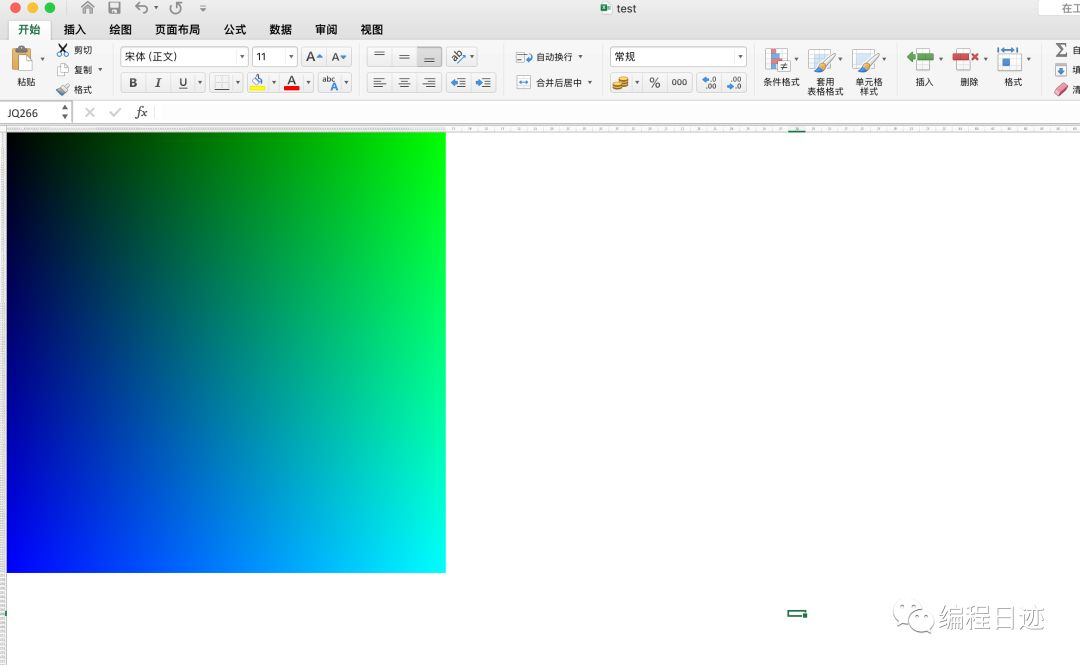用Python在Excel里画出蒙娜丽莎

• 时间:mona-lisa

PIL使用

PIL是Python里面做图像处理的时候十分常用的一个库，功能也是十分的强大，这里只需要用到PIL里一小部分的功能。

from PIL import Image
img = Image.open(img_path) # 读取图片
width, height = img.size # 获取图片大小
r, g, b = img.getpixel((w - 1, h - 1)) # 获取像素色彩值

Image.open()是PIL里面打开一张图片的函数，支持多种图片类型

img_path是图片路径，可以是相对路径，也可以是绝对路径

img.size是获取图片的size属性，包含图片的宽和高

img.getpixel()是获取图片色彩值的函数，需传入一个tuplelist，值为像素坐标xy

openpyxl使用

openpyxl几乎是Python里功能最全的操作excel文件的库了，这里也只需要用到它的一小部分功能。

import openpyxl
from openpyxl.styles import fills

workbook = openpyxl.Workbook()
worksheet = workbook.active
cell.fill = fills.PatternFill(fill_type="solid", fgColor=hex_rgb)
workbook.save(out_file)

openpyxl.Workbook()新建一个excel文件

workbook.active 激活一个工作表

cell.fill = fills.PatternFill(fill_type="solid", fgColor=hex_rgb)填充一个单元格，fill_type="solid"是填充类型，fgColor=hex_rgb是填充的颜色

workbook.save()保存文件，需传入要保存的文件名

写一段代码

1. getpixel()获取的颜色值是rgb十进制的，但fills.PatternFill 里的fgColor`参数接收到的颜色值是十六进制的值

这个问题其实就是十进制转十六进制，很容易解决

def int_to_16(num):
num1 = hex(num).replace('0x', '')
num2 = num1 if len(num1) > 1 else '0' + num1 # 位数只有一位的时候在前面补零
return num2

2. excel的单元格默认是长方形，修改为正方形才不会使图片变形

if h == 1:
_w = cell.column
_h = cell.col_idx
# 调整列宽
worksheet.column_dimensions[_w].width = 1

# 调整行高
worksheet.row_dimensions[h].height = 6

这里用到了双重for循环，外层是width，里层是height，是一列一列的填充颜色，因此判断if h == 1，避免多次调整列宽。

3. excel支持的样式数量有限

这个问题比较严重。如果直接对高清大图进行操作，最后输出的excel文件在打开的时候，可能会提示我们文件有问题，需要自动修复。

但是等它修复完成之后，会发现填充的所有颜色都消失了！MAX_WIDTH = 300
MAX_HEIGHT = 300
def resize(img):
w, h = img.size
if w > MAX_WIDTH:
h = MAX_WIDTH / w * h
w = MAX_WIDTH

if h > MAX_HEIGHT:
w = MAX_HEIGHT / h * w
h = MAX_HEIGHT
return img.resize((int(w), int(h)), Image.ANTIALIAS)

最终效果image-20191117175137916

全部代码

# draw_excel.py

from PIL import Image
import openpyxl
from openpyxl.styles import fills
import os

MAX_WIDTH = 300
MAX_HEIGHT = 300

def resize(img):
w, h = img.size
if w > MAX_WIDTH:
h = MAX_WIDTH / w * h
w = MAX_WIDTH

if h > MAX_HEIGHT:
w = MAX_HEIGHT / h * w
h = MAX_HEIGHT
return img.resize((int(w), int(h)), Image.ANTIALIAS)

def int_to_16(num):
num1 = hex(num).replace('0x', '')
num2 = num1 if len(num1) > 1 else '0' + num1
return num2

def draw_jpg(img_path):

img_pic = resize(Image.open(img_path))
img_name = os.path.basename(img_path)
out_file = './result/' + img_name.split('.') + '.xlsx'
if os.path.exists(out_file):
os.remove(out_file)

workbook = openpyxl.Workbook()
worksheet = workbook.active

width, height = img_pic.size

for w in range(1, width + 1):

for h in range(1, height + 1):
if img_pic.mode == 'RGB':
r, g, b = img_pic.getpixel((w - 1, h - 1))
elif img_pic.mode == 'RGBA':
r, g, b, a = img_pic.getpixel((w - 1, h - 1))

hex_rgb = int_to_16(r) + int_to_16(g) + int_to_16(b)

cell = worksheet.cell(column=w, row=h)

if h == 1:
_w = cell.column
_h = cell.col_idx
# 调整列宽
worksheet.column_dimensions[_w].width = 1
# 调整行高
worksheet.row_dimensions[h].height = 6

cell.fill = fills.PatternFill(fill_type="solid", fgColor=hex_rgb)

print('write in:', w, ' | all:', width + 1)
print('saving...')
workbook.save(out_file)
print('success!')

if __name__ == '__main__':
draw_jpg('mona-lisa.jpg')

附

count = 0
MAX_WIDTH = 255
for w in range(1, MAX_WIDTH + 1):
for h in range(1, MAX_WIDTH + 1):
cell = worksheet.cell(column=w, row=h)
if h == 1:
_w = cell.column
_h = cell.col_idx
# 调整列宽
worksheet.column_dimensions[_w].width = 1
# 调整行高
worksheet.row_dimensions[h].height = 6

if count < 255 ** 3:
back = int_to_16(num=count)
back = '0' * (6 - len(back)) + back
else:
back = ''.join([int_to_16(random.randint(0, 255)) for _ in range(3)])
cell.fill = fills.PatternFill(fill_type="solid", fgColor=back)
count += 1

count是记录颜色的变量，确保每一个颜色都不重复，但目前计算机RGB表示的颜色最多只有256^3种...还有点好看。！？？